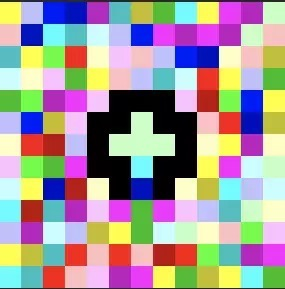17611538698
developer@21cto.com

五个怪异难懂的编程语言

1、Piet

Piet是一种用颜色制作的编程语言。2、莎士比亚

Variable, Definition

Act I: Hamlet's insults and flattery.

Scene I: The insulting of Romeo.

Juliet:

Am I better than you?

Hamlet:

If so, let us proceed to scene III.

3、空格

T    L

S S S S S T    T    S S T    S T    L

T    L

S S S S S T    T    S T    T    S S L

T    L

S S S S S T    T    S T    T    S S L

T    L

S S S S S T    T    S T    T    T    T    L

T    L

S S S S S T    S T    T    S S L

T    L

S S S S S T    S S S S S L

T    L

S S S S S T    T    T    S T    T    T    L

T    L

S S S S S T    T    S T    T    T    T    L

T    L

S S S S S T    T    T    S S T    S L

T    L

S S S S S T    T    S T    T    S S L

T    L

S S S S S T    T    S S T    S S L

T    L

S S S S S T    S S S S T    L

T    L

S S L

L

L

4、JSF*ck

JSF*ck是基于JavaScript的语言，它仅使用方括号和圆括号以及感叹号和加号，也就是正则表达式的语法。

(+[![]]+[+(+!+[]+(!+[]+[])[!+[]+!+[]+!+[]]+(+!+[])+(+[])+(+[])+(+[]))])[+!+[]+[+[]]]

// Returns y

(![]+[])[+!![]]

// Returns a

!+[]+!+[]+!+[]+!+[]+!+[]+!+[]+!+[]+!+[]+!+[]

// Returns 9

5.Malbolge

Malbolge中的大多数代码是使用其他语言生成的，尽管有些脚本是用纯Malbolge编写的。下面显示了如何在Malbolge中输出“ Hello World”的示例：

(=<`#9]~6ZY32Vx/4Rs+0No-&Jk)"Fh}|Bcy?`=*z]Kw%oG4UUS0/@-ejc(:'8dc

评论